# How to Replace Formulas with Their Values in Excel

This post will guide you how to replace formulas with their calculated values in Excel. How do I replace all formulas with their values using VBA Macro in Excel.

Assuming that you have a list of data that contain formulas in range B1:B4, and you want to replace all formulas with their calculated values, how to do it. You can use the Paste Special command or an Excel VBA Macro to achieve the result.

## Replace Formulas with Their Values

If you want to replace all formulas in the selected range of cells with their values in Excel, you can do the following steps:

#1 select the range of cells that contain formulas. And press Ctrl + C keys on your keyboard.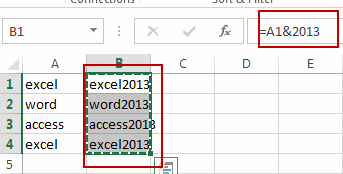#2 right click on the selected cells, and select the Paste Values menu under the Paste Options from the popup menu list.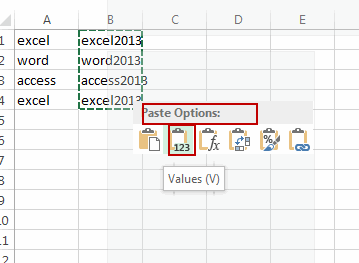#3 you would notice that all the formulas have been replaced with their calculated results in the selected range.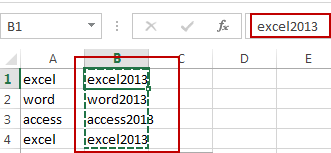## Replace Formulas with Their Values Using VBA

You can also use an Excel VBA Macro to achieve the same result of replacing all formulas with their calculated values. Just do the following steps:

#1 open your excel workbook and then click on “Visual Basic” command under DEVELOPER Tab, or just press “ALT+F11” shortcut.#2 then the “Visual Basic Editor” window will appear.

#3 click “Insert” ->”Module” to create a new module.#4 paste the below VBA code  into the code window. Then clicking “Save” button.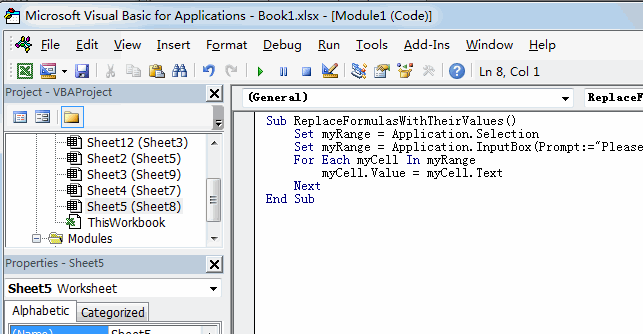#5 back to the current worksheet, then run the above excel macro. Click Run button.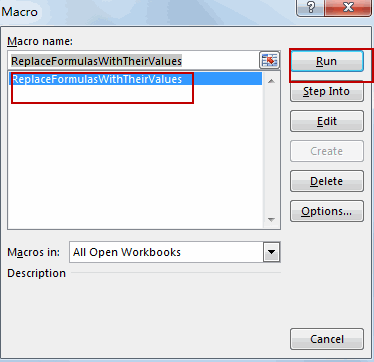#6 Please select one range that contain formuals. Click OK button.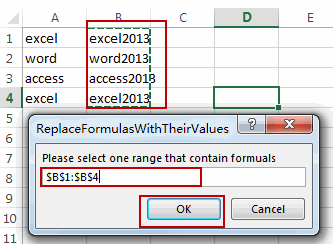#7 Let’s see the result: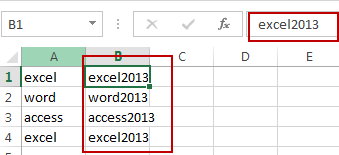### You might also like:

Sidebar